Physics 2102 Gabriela Gonzlez Physics 2102 Electric fields

• Slides: 22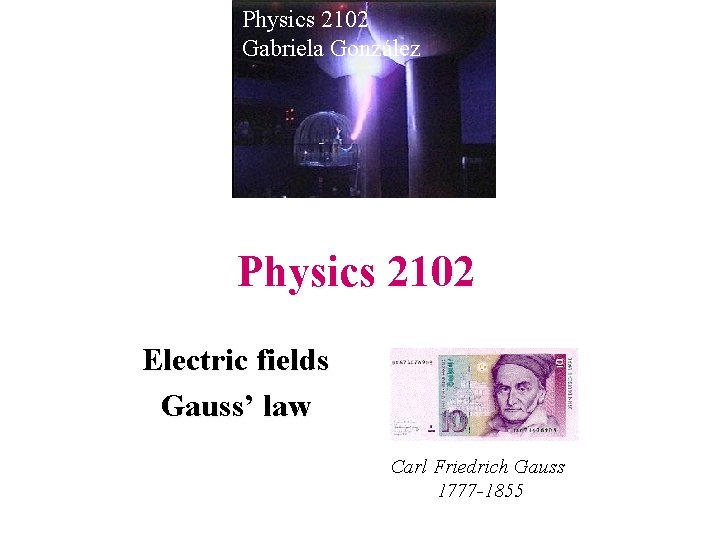Physics 2102 Gabriela González Physics 2102 Electric fields Gauss’ law Carl Friedrich Gauss 1777 -1855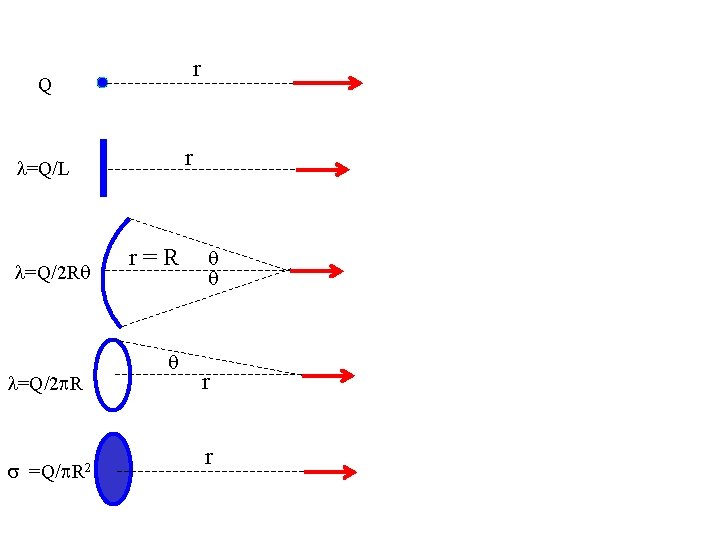r Q r l=Q/L l=Q/2 Rq l=Q/2 p. R s =Q/p. R 2 r=R q q q r r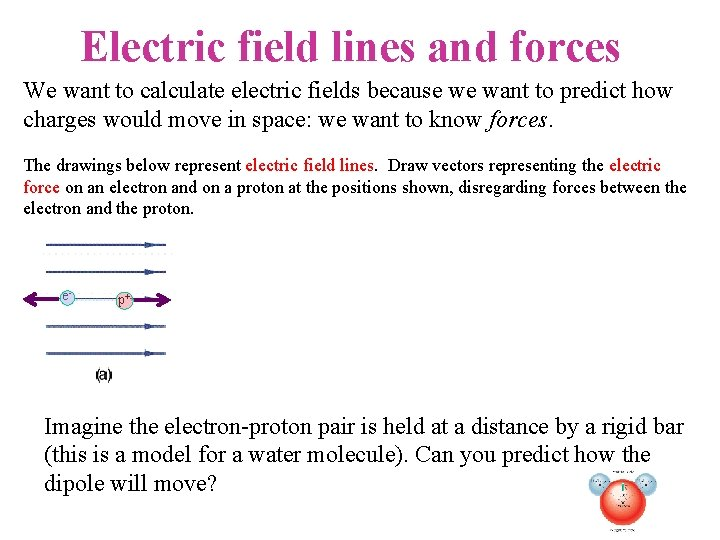Electric field lines and forces We want to calculate electric fields because we want to predict how charges would move in space: we want to know forces. The drawings below represent electric field lines. Draw vectors representing the electric force on an electron and on a proton at the positions shown, disregarding forces between the electron and the proton. e- p+ (d) Imagine the electron-proton pair is held at a distance by a rigid bar (this is a model for a water molecule). Can you predict how the dipole will move?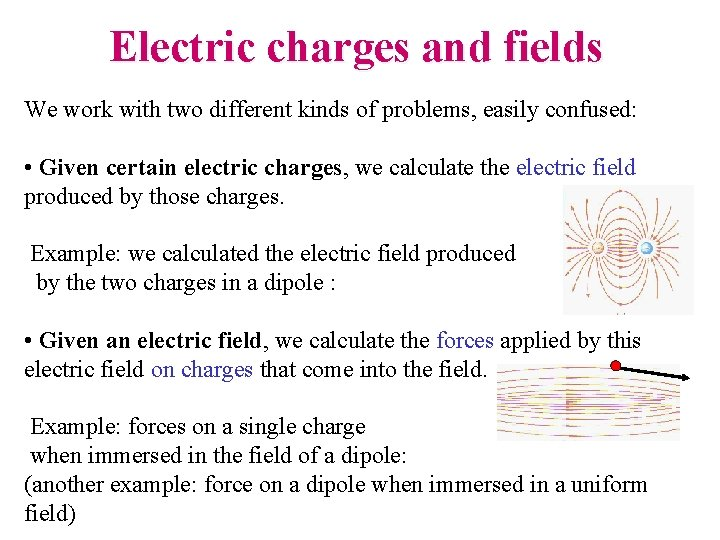Electric charges and fields We work with two different kinds of problems, easily confused: • Given certain electric charges, we calculate the electric field produced by those charges. Example: we calculated the electric field produced by the two charges in a dipole : • Given an electric field, we calculate the forces applied by this electric field on charges that come into the field. Example: forces on a single charge when immersed in the field of a dipole: (another example: force on a dipole when immersed in a uniform field)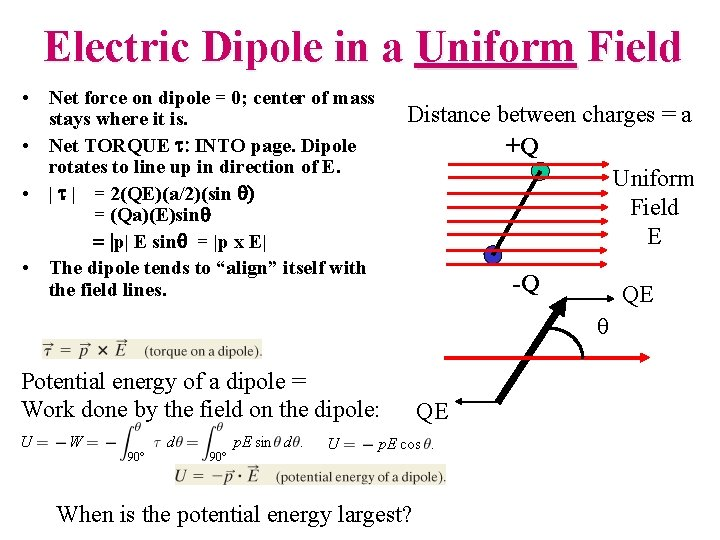Electric Dipole in a Uniform Field • Net force on dipole = 0; center of mass stays where it is. • Net TORQUE t: INTO page. Dipole rotates to line up in direction of E. • | t | = 2(QE)(a/2)(sin q) = (Qa)(E)sinq = |p| E sinq = |p x E| • The dipole tends to “align” itself with the field lines. Distance between charges = a +Q Uniform Field E -Q QE q Potential energy of a dipole = Work done by the field on the dipole: When is the potential energy largest? QE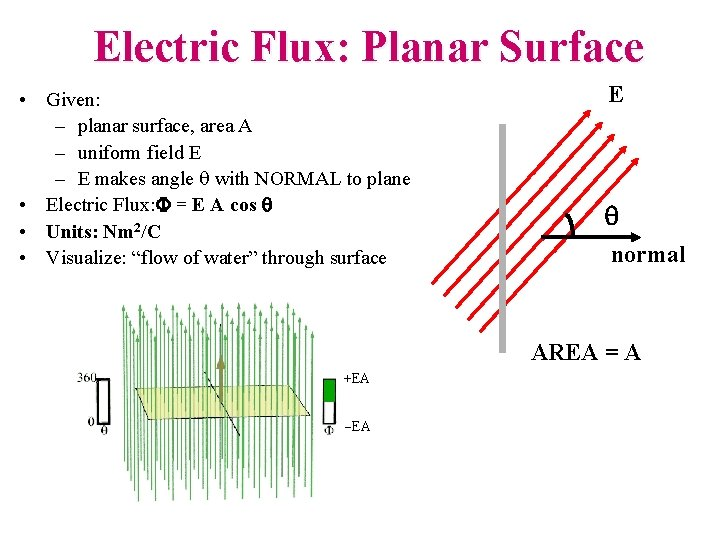Electric Flux: Planar Surface • Given: – planar surface, area A – uniform field E – E makes angle q with NORMAL to plane • Electric Flux: F = E A cos q • Units: Nm 2/C • Visualize: “flow of water” through surface E q normal AREA = A +EA -EA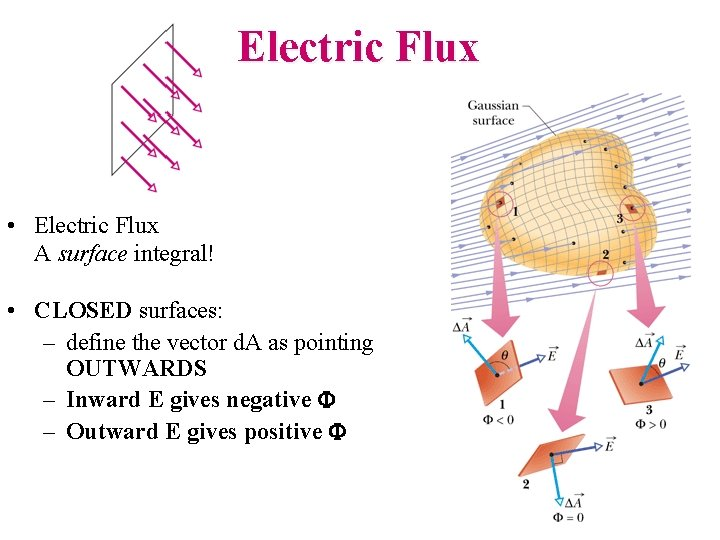Electric Flux • Electric Flux A surface integral! • CLOSED surfaces: – define the vector d. A as pointing OUTWARDS – Inward E gives negative F – Outward E gives positive F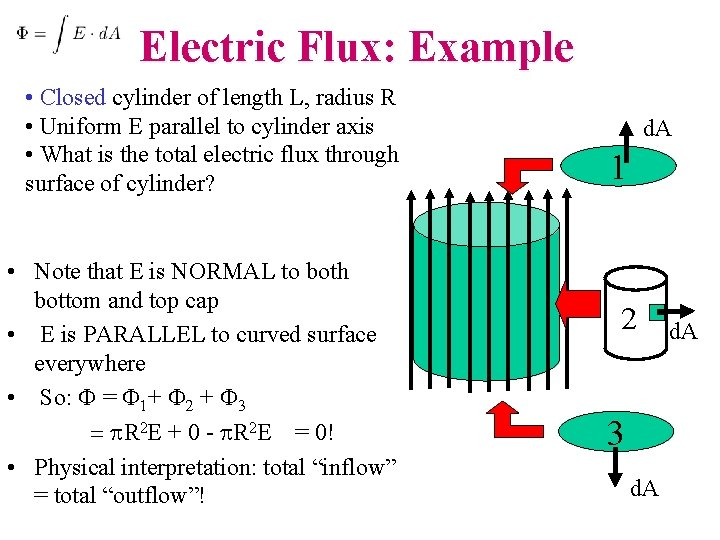Electric Flux: Example • Closed cylinder of length L, radius R • Uniform E parallel to cylinder axis • What is the total electric flux through surface of cylinder? • Note that E is NORMAL to both bottom and top cap • E is PARALLEL to curved surface everywhere • So: F = F 1+ F 2 + F 3 = p. R 2 E + 0 - p. R 2 E = 0! • Physical interpretation: total “inflow” = total “outflow”! d. A 1 2 3 d. AElectric Flux: Example • • Spherical surface of radius R=1 m; E is RADIALLY INWARDS and has EQUAL magnitude of 10 N/C everywhere on surface What is the flux through the spherical surface? (a) -(4/3)p. R 2 E = -13. 33 p Nm 2/C (b) 4 p. R 2 E = +40 p Nm 2/C (c) 4 p. R 2 E= -40 p Nm 2/C What could produce such a field? What is the flux if the sphere is not centered on the charge?Gauss’ Law • Consider any ARBITRARY CLOSED surface S -- NOTE: this does NOT have to be a “real” physical object! • The TOTAL ELECTRIC FLUX through S is proportional to the TOTAL CHARGE ENCLOSED! • The results of a complicated integral is a very simple formula: it avoids long calculations! S (One of Maxwell’s 4 equations)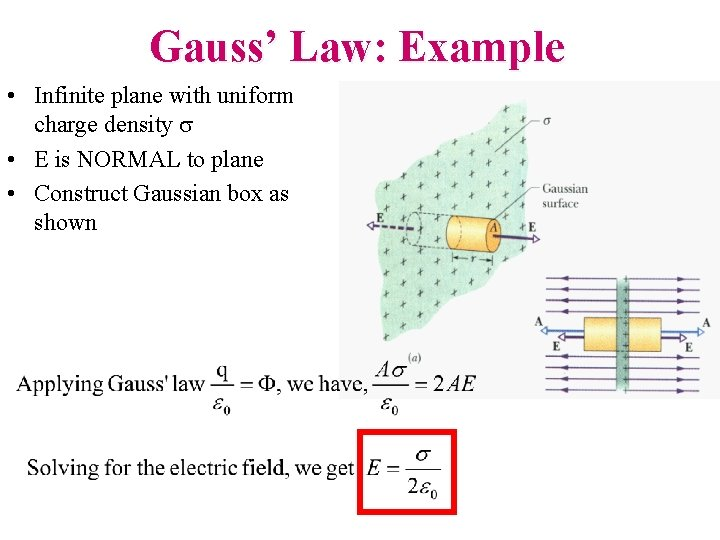Gauss’ Law: Example • Infinite plane with uniform charge density s • E is NORMAL to plane • Construct Gaussian box as shown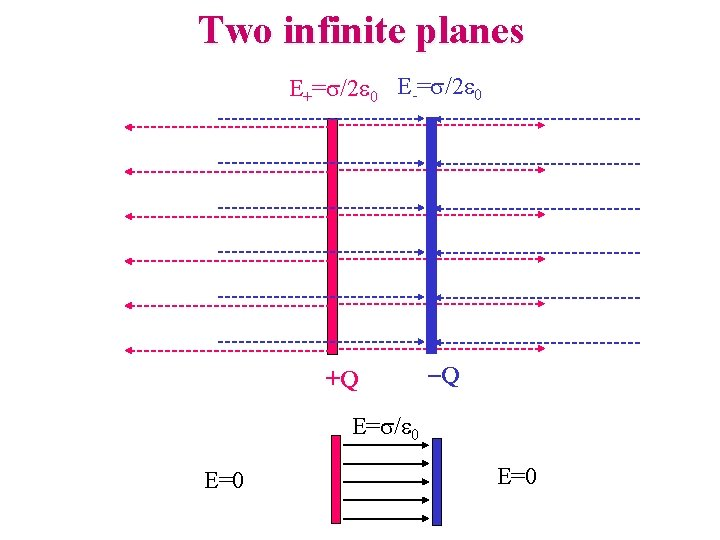Two infinite planes E+=s/2 e 0 E-=s/2 e 0 +Q -Q E=s/e 0 E=0Gauss’ Law: Example Cylindrical symmetry • Charge of 10 C is uniformly spread over a line of length L = 1 m. • Use Gauss’ Law to compute magnitude of E at a perpendicular distance of 1 mm from the center of the line. • Approximate as infinitely long line -- E radiates outwards. • Choose cylindrical surface of radius R, length L co-axial with line of charge. E=? 1 m R = 1 mm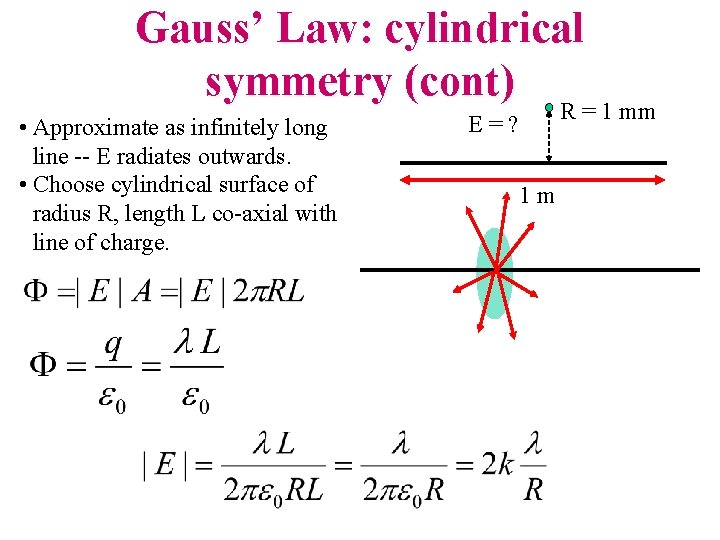Gauss’ Law: cylindrical symmetry (cont) R = 1 mm • Approximate as infinitely long line -- E radiates outwards. • Choose cylindrical surface of radius R, length L co-axial with line of charge. E=? 1 mCompare with last class! if the line is infinitely long (L >> a)…Gauss’ Law: Example Spherical symmetry • Consider a POINT charge q & pretend that you don’t know Coulomb’s Law • Use Gauss’ Law to compute the electric field at a distance r from the charge • Use symmetry: – draw a spherical surface of radius R centered around the charge q – E has same magnitude anywhere on surface – E normal to surface r q EGauss’ Law: Example • A spherical conducting shell has an excess charge of +10 C. • A point charge of -15 C is located at center of the sphere. • Use Gauss’ Law to calculate the charge on inner and outer surface of sphere (a) Inner: +15 C; outer: 0 (b) Inner: 0; outer: +10 C (c) Inner: +15 C; outer: -5 C R 2 R 1 -15 CGauss’ Law: Example • Inside a conductor, E = 0 under static equilibrium! Otherwise electrons would keep moving! • Construct a Gaussian surface inside the metal as shown. (Does not have to be spherical!) • Since E = 0 inside the metal, flux through this surface = 0 • Gauss’ Law says total charge enclosed = 0 • Charge on inner surface = +15 C -5 C Since TOTAL charge on shell is +10 C, Charge on outer surface = +10 C - 15 C = -5 C! +15 C -15 CSummary: • Gauss’ law: F = E d. A provides a very direct way to compute the electric flux if we know the electric field. • In situations with symmetry, knowing the flux allows us to compute the fields reasonably easily.Electric field of a ring Let’s calculate the field produced by a ring of radius R with total charge +Q, on a point on the axis, at a distance z from the center. A differential ring element will have charge dq, and will produce a field d. E with direction as shown in the figure. The magnitude of the field is d. E=kdq/r 2. Notice that the distance r is the same for all elements! By symmetry, we know the field will point up, so we will only need to integrate the component d. Ey=d. Ecosq= (k dq/r 2)(z/r)=k(z/r 3)dq. Notice that the angle q is the same for all elements, it is not an integration variable! We integrate over the ring to get the magnitude of the total field: E = ∫d. Ey = ∫k(z/r 3)dq= k(z/r 3) ∫dq = k. Qz/r 3 = k. Qz/(R 2+z 2)3/2 No integral table needed! What’s the field very far from the ring? If z>>R, E~k. Qz/z 3=k. Q/z 2 : of course, the field of a point charge Q.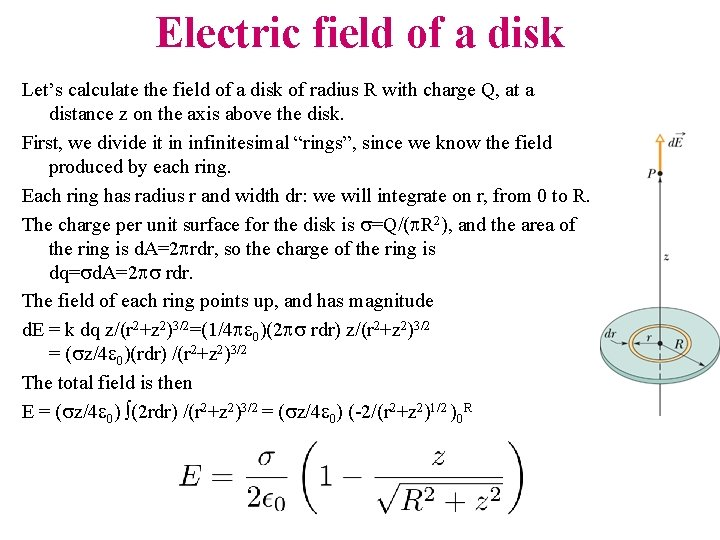Electric field of a disk Let’s calculate the field of a disk of radius R with charge Q, at a distance z on the axis above the disk. First, we divide it in infinitesimal “rings”, since we know the field produced by each ring. Each ring has radius r and width dr: we will integrate on r, from 0 to R. The charge per unit surface for the disk is s=Q/(p. R 2), and the area of the ring is d. A=2 prdr, so the charge of the ring is dq=sd. A=2 ps rdr. The field of each ring points up, and has magnitude d. E = k dq z/(r 2+z 2)3/2=(1/4 pe 0)(2 ps rdr) z/(r 2+z 2)3/2 = (sz/4 e 0)(rdr) /(r 2+z 2)3/2 The total field is then E = (sz/4 e 0) ∫(2 rdr) /(r 2+z 2)3/2 = (sz/4 e 0) (-2/(r 2+z 2)1/2 )0 RElectric field of a disk If we are very far from the disk, z>>R, E~0: of course, it gets vanishing small with distance. If we use We get E ~ (s/4 e 0)(R 2/z 2) = (Q/p. R 2)/(4 e 0)(R 2/z 2) =k. Q/z 2. (Of course!) If the disk is very large (or we are very close), R>>z, and E~s/2 e 0 The field produced by any large charged surface is a uniform field, with magnitude s/2 e 0.